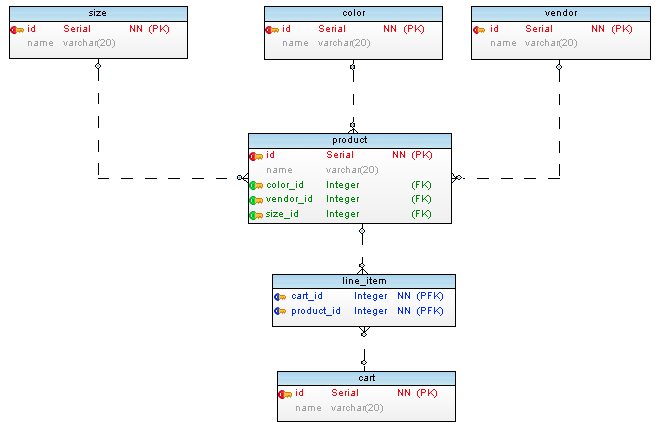## O2MRelation

Kind: global class

### new O2MRelation(args)

Class which represent one to many relationship which resembles `hasMany` relation in ORMs (Object Relational Mappers). Provides attributes and methods for details of the relationship.

Below is a database schema as an example:

``````size -------------------
id (PK)                |  ---------------------------< line_item >------------ cart
name                   |  |                            product_id (PFK)        id (PK)
|  |                            cart_id    (PFK)        name
^  |
color -------------< product >------------- vendor
id (PK)              id        (PK)         id (PK)
name                 name                   name
color_id  (FK)
size_id   (FK)
vendor_id (FK)

``````

Below is the same schema as image:Some definitions used in descriptions for O2MRelation. Source Table: Table which this relationship belongs to. Target Table: Table that is related to base table.

Param Type Description
args `Object` Attributes of the O2MRelation instance to be created.
args.sourceTable `Table` Source Table which this relation belongs to.
args.targetTable `Table` Target Table which this relation is referring to.
args.constraint `Constraint` Foreign key constraint between source table and target table.

Example

``````// Example tables have single primary key and examples first relation. So zero index () is used. Use all array elements if necessary.
// product ----< line_item
// (source)       (target)

let relation         = product.o2mRelations;              // RELATION:    product ---< line_item
let constraint       = relation.constraint;                  // CONSTRAINT:           ^-- product_has_carts
let sourceTable      = relation.sourceTable;                 // TABLE:       product
let targetTable      = relation.targetTable;                 // TABLE:       line_item
let FKColumn         = relation.constraint.columns;       // COLUMN:      product_id  (from line_item table)
let sourcePKColumn   = relation.sourceTable.primaryKeys;  // COLUMN:      id          (from product table)
``````

### o2MRelation.type : `relationType`

Type of relation which is `ONE TO MANY`.

Kind: instance property of `O2MRelation`

### o2MRelation.sourceTable : `Table`

Table which this relation belongs to.

Kind: instance property of `O2MRelation`
Example

``````let relation     = product.O2MRelationRelations;  // RELATION:    product ---< line_item
let sourceTable  = relation.sourceTable;             // TABLE:       product
``````

### o2MRelation.targetTable : `Table`

Table which this relation is referring to.

Kind: instance property of `O2MRelation`
Example

``````let relation     = product.O2MRelationRelations;  // RELATION:    product ---< line_item
let targetTable  = relation.targetTable;             // TABLE:       line_item
``````

### o2MRelation.constraint : `Table`

Foreign key constraint between source table and target table.

Kind: instance property of `O2MRelation`
Example

``````let relation     = product.O2MRelationRelations;  // RELATION:    product ---< line_item
let constraint   = relation.constraint;              // CONSTRAINT:           ^-- product_has_carts
let FKColumn     = relation.constraint.columns;   // COLUMN:      product_id (from line_item table)
``````

### o2MRelation.generateName([strategy]) ⇒ `string`

(! EXPERIMENTAL) Returns name for relation using given strategy. Please see Relation Names for details.

Kind: instance method of `O2MRelation`
Returns: `string` - - Relation name.
See: Relation Names

Param Type Description
[strategy] `string` (simple, complex) Naming strategy to use.